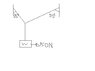# Equilibrium Problem(Tension of each cord)

• durty53

#### durty53

I have a problem..I cannot solve this one..the angles are on the side.. I don't have any idea..any answers would be much appreciated!

#### Attachments

•science.JPG
5.2 KB · Views: 451
Indicate the forces acting. Apply the conditions for equilibrium.

the weight(W) of teh suspended body is 150N

the weight(W) of teh suspended body is 150N
OK.

Consider the forces acting at the connection point. Name them.

The book said that I should find the tension in each cord if the weight(W) of the suspended body is 150N

The book said that I should find the tension in each cord if the weight(W) of the suspended body is 150N
Yes. Label the cord tensions T1 and T2.

Since there's equilibrium, what must be the net force?

Yes. Label the cord tensions T1 and T2.

Since there's equilibrium, what must be the net force?

the net force must be zero

the net force must be zero
Good!

Now express that mathematically. Write equations for the vertical and horizontal force components.